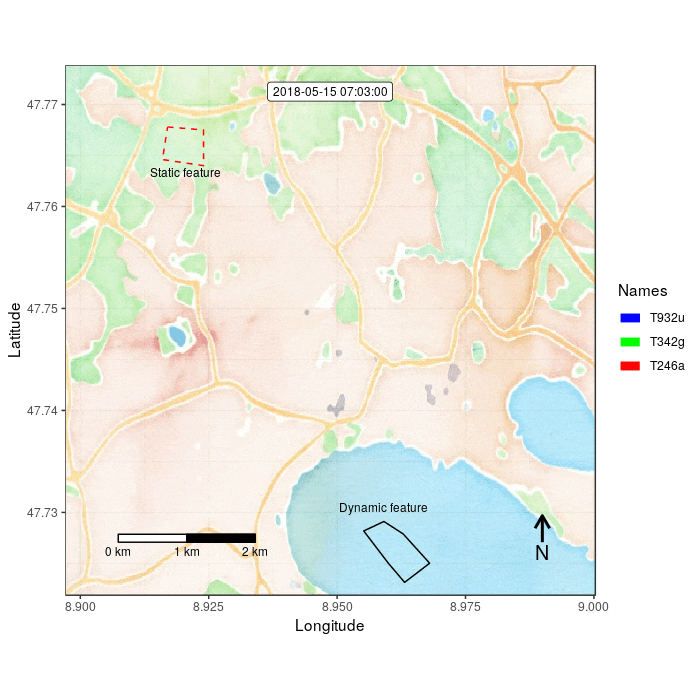moveVis is entierly based on the ggplot2 grammar of graphics. Each list element in frames is a ggplot2 object that represents a single animation frame. Thus, it is possible to customize each frame individually using ggplot2 functions. Instead, moveVis provides a set of functions for making it simpler to cutomize frames. We will use some of them in the following to customize frames that we created in the prior section:

library(moveVis)
library(move)
library(raster)
library(ggplot2)

data("move_data")

# align movement tracks
move_data <- align_move(move_data, res = 4, unit = "mins")

# create frames
frames <- frames_spatial(move_data, path_colours = c("red", "green", "blue"),
map_service = "osm", map_type = "watercolor", alpha = 0.5)

# edit frames
frames <- add_labels(frames, x = "Longitude", y = "Latitude") # add labels, e.g. axis labels
frames <- add_timestamps(frames, move_data, type = "label") # add timestamps

Alternatively, use the pipe, which (in my opinion) makes this more elegant:

# edit frames
frames <- add_labels(frames, x = "Longitude", y = "Latitude") %>%

## Have a look at one of the frames:
frames[]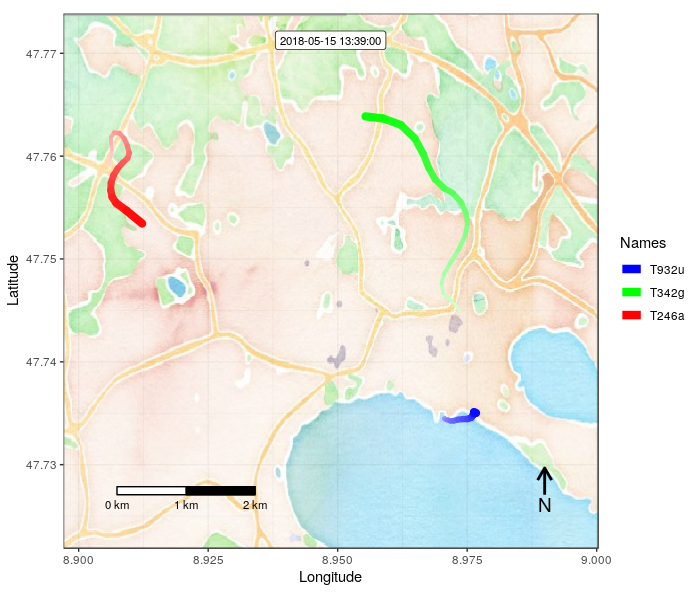For further details on these functions, please see their help files. If you want to apply your own ggplot2 syntax to frames, e.g. for drawing polygons, lines or points that are static or even change with time, you can do this frame-wise. In the following example, we customize one individual frame just as if you would work with a single ggplot2 object:

data <- data.frame(x = c(8.917, 8.924, 8.924, 8.916, 8.917),
y = c(47.7678, 47.7675, 47.764, 47.7646, 47.7678))

# just customize a single frame and have a look at it
frame_test <- frames[] + geom_path(aes(x = x, y = y), data = data,
colour = "red", linetype = "dashed")
frame_test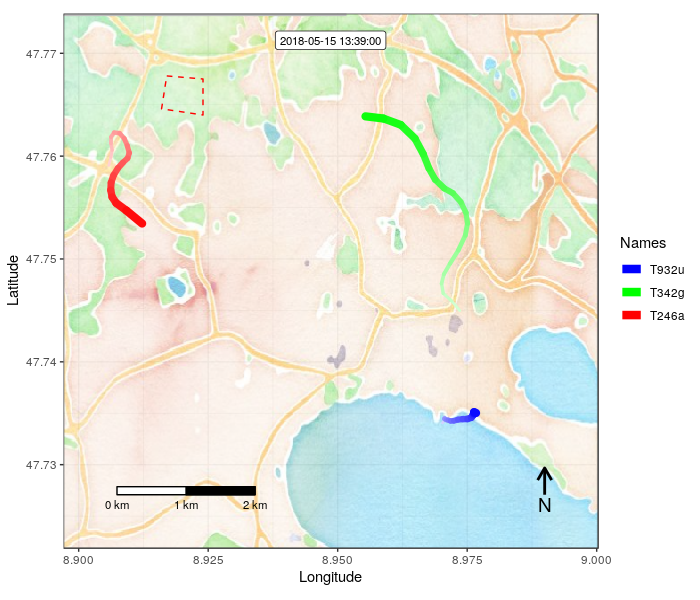If you just want to change one or a small selection of frames, you could just manipulate those frames like shown above and assign the cusomized ggplot2 frames to the equivalent elements in your frames list.

If you want to edit all frames, you can use the add_gg() function. Here, we want to mark a field on the map on all frames. For this, we use the geom_path() function of ggplot2 with add_gg():

# or customize all frames at once using add_gg:
frames = add_gg(frames, gg = expr(geom_path(aes(x = x, y = y), data = data,
colour = "red", linetype = "dashed")), data = data)

The field marking is now added to all frames. Let’s add some text to describe the field marking:

frames <- add_text(frames, "Static feature", x = 8.9205, y = 47.7633,
colour = "black", size = 3)

## Have a look at one of the frames:
frames[]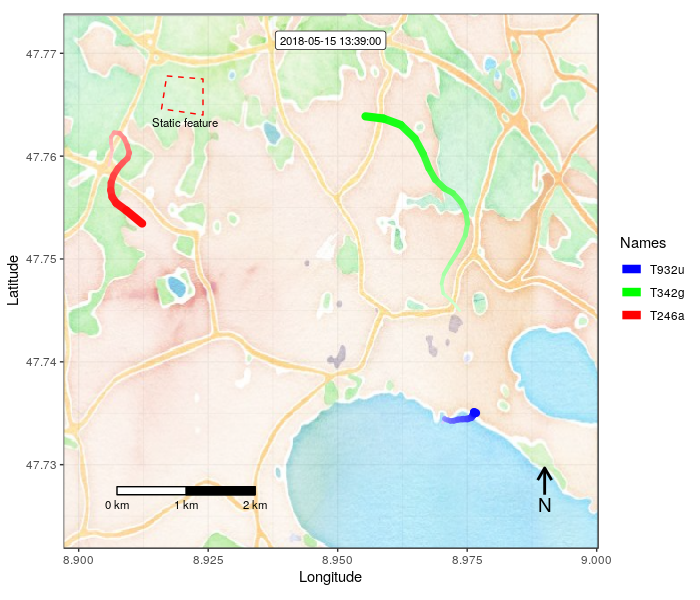add_gg() can also be used to customize each frame consecutively, e.g. to add dynamic marks that move or change with time. Both arguments gg and data can take lists of the same length as frames. If one of these arguments or both are lists, each list element is applied to the according element in frames. Let’s add a another field mark that is slightly changing with each frame:

## create data.frame containing corner coordinates
data <- data.frame(x = c(8.96, 8.955, 8.959, 8.963, 8.968, 8.963, 8.96),
y = c(47.725, 47.728, 47.729, 47.728, 47.725, 47.723, 47.725))
## make a list from it by replicating it by the length of frames
data <- rep(list(data), length.out = length(frames))

## now alter the coordinates to make them shift
data <- lapply(data, function(x){
y <- rnorm(nrow(x)-1, mean = 0.00001, sd = 0.0001)
x + c(y, y)
})

## draw each individual polygon to each frame
frames = add_gg(frames, gg = expr(geom_path(aes(x = x, y = y), data = data,
colour = "black")), data = data)

frames[]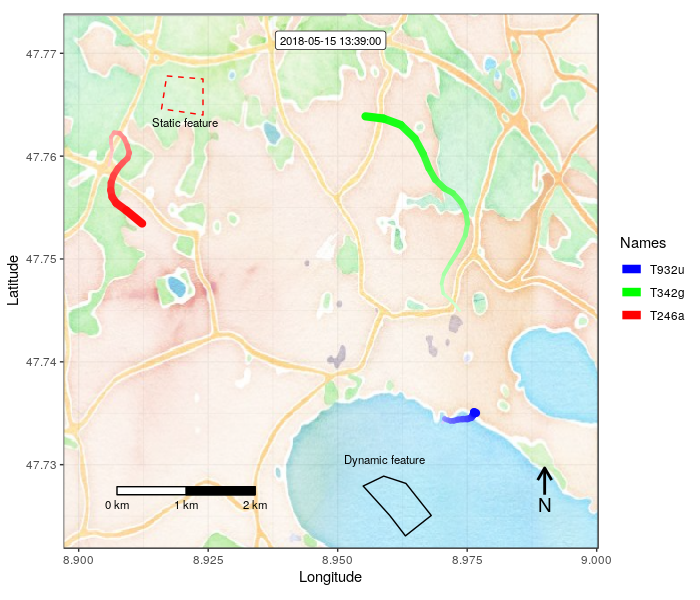Animate the the customized frames as we did in the prior section using animate_frames().
animate_frames(frames, "/full/path/to/example_2.gif")